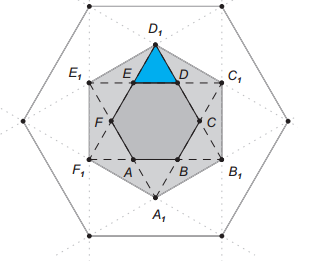# Zero In On A Kaleidoscope

Geometry Level 1Extending the sides of a regular hexagon $ABCDEF$, of an area of $1\text{ cm}^2$, we get another hexagon $A_1B_1C_1D_1E_1F_1$.

Repeating this process, we can get other hexagons $A_2B_2C_2D_2E_2F_2$ etc.

What is the area of the blue triangle?

×